Next: Worked Examples Up: Capacitance Previous: Capacitors in Series and

## Energy Stored by Capacitors

Let us consider charging an initially uncharged parallel plate capacitor by transferring a charge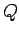from one plate to the other, leaving the former plate with charge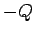and the later with charge. Of course, once we have transferred some charge, an electric field is set up between the plates which opposes any further charge transfer. In order to fully charge the capacitor, we must do work against this field, and this work becomes energy stored in the capacitor. Let us calculate this energy.

Suppose that the capacitor plates carry a chargeand that the potential difference between the plates is. The work we do in transferring an infinitesimal amount of charge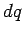from the negative to the positive plate is simply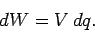(117)

In order to evaluate the total workdone in transferring the total chargefrom one plate to the other, we can divide this charge into many small increments, find the incremental workdone in transferring this incremental charge, using the above formula, and then sum all of these works. The only complication is that the potential differencebetween the plates is a function of the total transferred charge. In fact,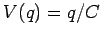, so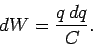(118)

Integration yields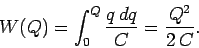(119)

Note, again, that the work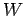done in charging the capacitor is the same as the energy stored in the capacitor. Since, we can write this stored energy in one of three equivalent forms: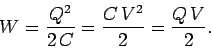(120)

These formulae are valid for any type of capacitor, since the arguments that we used to derive them do not depend on any special property of parallel plate capacitors.

Where is the energy in a parallel plate capacitor actually stored? Well, if we think about it, the only place it could be stored is in the electric field generated between the plates. This insight allows us to calculate the energy (or, rather, the energy density) of an electric field.

Consider a vacuum-filled parallel plate capacitor whose plates are of cross sectional area, and are spaced a distanceapart. The electric fieldbetween the plates is approximately uniform, and of magnitude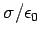, where, andis the charge stored on the plates. The electric field elsewhere is approximately zero. The potential difference between the plates is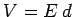. Thus, the energy stored in the capacitor can be written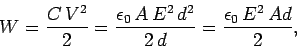(121)

where use has been made of Eq. (108). Now,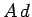is the volume of the field-filled region between the plates, so if the energy is stored in the electric field then the energy per unit volume, or energy density, of the field must be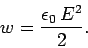(122)

It turns out that this result is quite general. Thus, we can calculate the energy content of any electric field by dividing space into little cubes, applying the above formula to find the energy content of each cube, and then summing the energies thus obtained to obtain the total energy.

It is easily demonstrated that the energy density in a dielectric medium is(123)

where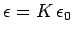is the permittivity of the medium. This energy density consists of two elements: the energy density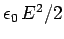held in the electric field, and the energy densityheld in the dielectric medium (this represents the work done on the constituent molecules of the dielectric in order to polarize them).Next: Worked Examples Up: Capacitance Previous: Capacitors in Series and
Richard Fitzpatrick 2007-07-14